# Excel TODAY function to insert today's date and more

The tutorial shows how to insert today's date in your worksheets and demonstrates a few other uses of TODAY function in Excel.

You want to put today's date in some cell? Or you wish to highlight the current date in your Excel calendar? Or you'd like to find the date closest to today? All this can be done by using the Excel TODAY function and this tutorial will teach you how.

## Excel TODAY function - syntax and basic uses

The TODAY function in Excel does exactly what its name indicates - returns today's date.

The syntax of the TODAY function is as simple as it could possible be - it does not have any arguments at all. Whenever you need to insert today's date in Excel, just enter the following formula in a cell:

`=TODAY()`

You can format the value returned by TODAY in any built-in or custom date format. For example, this way: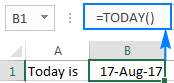### 4 things you should know about TODAY in Excel

1. TODAY() is a volatile function, which means that it updates itself continuously every time a worksheet is opened or changed.
2. If a TODAY formula does not update automatically, most likely automatic recalculation is turned off in your workbook. To turn it on again, go to the Formulas tab > Calculation Options, and select Automatic.
3. To enter today's date in Excel as a static unchangeable value, use these keyboard shortcuts.
4. If you want to insert current date and time, use the NOW function instead of TODAY.

## How to insert today's date in Excel

There are two ways to enter the current date in Excel - a formula and shortcut. Which one to use depends on whether you want a static or dynamic value.

### Excel formula for today's date

The value returned by the TODAY function updates automatically, so the below formula is useful if you want the worksheet to always display the current date, regardless of when you open it.

`=TODAY()`

To clarify what kind of date that is, you can concatenate TODAY() with some explanatory text, for example:

`=CONCATENATE("Today is ",TEXT(TODAY(), "mmmm dd, yyyy"))`

Because in the internal Excel system dates and times are stored as numbers, concatenating text with the TODAY() formula directly would result in a meaningless string like "Today is 42965". To avoid this, we nest Excel's TODAY function within the TEXT function to display the date in the desired format.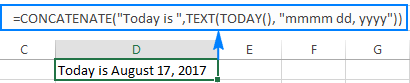### Shortcuts to get today's date in Excel

The inset today's date as an unchangeable timestamp that won't automatically update the next day, use one of the following keyboard shortcuts:

• To inset today's date: Ctrl + ;
• To insert the current time: Ctrl + Shift + ;
• To enter the current date and time: Ctrl + ; then Space and then Ctrl + Shift + ;

## How to use TODAY function in Excel - formula examples

As you already know, the main purpose of the Excel TODAY function is to get today's date. Aside from that, you can use TODAY() in combination with other functions to perform more complex calculations based on the current date. Below you will find a few examples of such formulas.

### Add or subtract days to/from today's date

To add or subtract a specific number of days to/from the current date, use a simple arithmetic operation of addition or subtraction, respectively.

For example, to add 7 days to todays' date, use this formula:

=TODAY()+7

To subtract 7 days from the current date, go with this one:

=TODAY()-7

To exclude weekends (Saturday and Sunday) from your calculations, nest the TODAY function within WORKDAY that deals with weekdays only:

To add 7 workdays to today's date:

=WORKDAY(TODAY(), 7)

To subtract 7 workdays from today's date:

=WORKDAY(TODAY(), -7)

The following screenshot shows the results: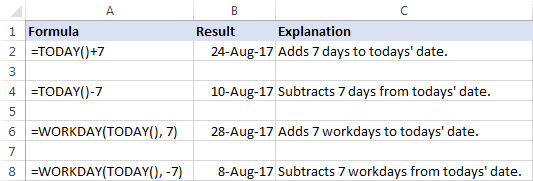Tip. To display the calculated date correctly, set the formula cell's format to Date.

### Get the number of days before or after a certain date

To calculate how many days are left before some data, subtract today's date from the future date you are counting toward:

date-TODAY()

The date can be supplied directly to a formula in the format that Excel can understand, or by using the DATE function, or as a reference to the cell containing the date.

For example, to find out how many days are left till December 31, 2017, use one of these formulas:

`=A2-TODAY()`

`=DATE(2017,12,31)-TODAY()`

`="12/31/2017"-TODAY()`

All three formulas tell us that at the moment of writing (August 17, 2017), 136 days were left till the end of the year 2017: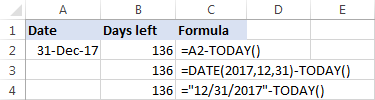To calculate the number of days since a certain date, subtract the past date from today's date:

TODAY()-date

For example, to find out how many days have passed since January 1, 2017, use one of these formulas:

`=TODAY()-A2`

`=TODAY()-DATE(2017,1,1)`

`=TODAY()-"1/1/2017"`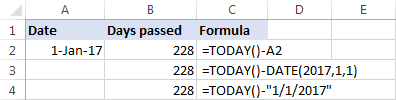Tip. If the result is not displayed correct, be sure to apply the General format to the formula cell.

### Calculate months since or before a certain date

To get the number of months between today and a past date, use the DATEDIF(start_date, end_date, unit) function with the past date in the start_date argument, TODAY() as end_date and "m" unit denoting months:

DATEDIF(past_ date, TODAY(),"m")

To get the number of months between today and a future date, swap the start_date and end_date arguments:

DATEDIF(TODAY(), future-date,"m")

With the date of interest in cell A4, use the following formulas to calculate time difference in the number of complete months:

To calculate months since a certain date in the past:

`=DATEDIF(A4,TODAY(),"m")`

To calculate months before a certain date in the future:

`=DATEDIF(TODAY(),A4,"m")`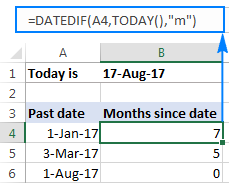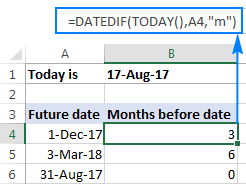### Calculate years since / before a certain date

The formulas to calculate years based on today's date are similar to the ones discussed in the above example. The difference is that you use "y" unit to get the number of complete years between today and another data:

To calculate years since a past date:

DATEDIF(past_ date, TODAY(),"y")

To calculate years before a future date:

DATEDIF(TODAY(), future-date,"y")

Assuming the past/future date is in cell A4, you should be good with using these formulas:

The number of complete years since date:

`=DATEDIF(A4,TODAY(),"y")`

The number of complete years before date:

`=DATEDIF(TODAY(),A4,"y")`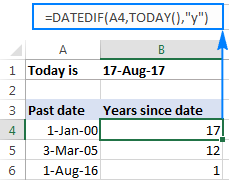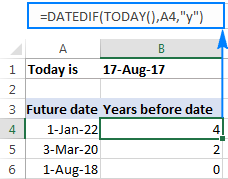### Get age from birthdate

If you know someone's year of birth, you can subtract that year from the current year to find the person's age:

YEAR( TODAY())-year_of_birth

For example, if the person was born in 2000, you use the following formula to get his/her age:

`=YEAR( TODAY())-2000`

Or, you can enter the year of birth in a separate cell and reference that cell in your formula: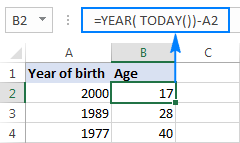You can learn a few other age calculation formulas in this tutorial: How to get age from date of birth in Excel.

### Find the nearest date to today

If you are curious to know which date in a list is closest to today's date, use one of the following array formulas to find it out.

#### Get a past date closest to today

To find a past date nearest to the current date, first "filter out" the dates greater than today, and then use the MAX function to get the largest date among the remaining ones:

MAX(IF(range < TODAY(), range))

With the dates in cells A2 to A10, the formula would go as follows:

`=MAX(IF(\$A\$2:\$A\$10 < TODAY(), \$A\$2:\$A\$10))`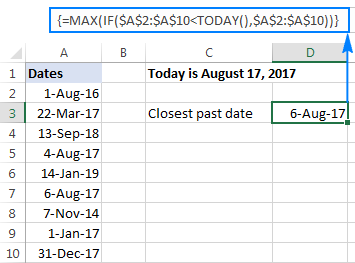#### Get a future date closest to today

To find the nearest future date, identify the dates that are greater than today, and then use the MIN function to get the smallest date among them:

MIN(IF(range >TODAY(), range))

For our sample data set, we'd use this formula:

`=MIN(IF(\$A\$2:\$A\$10 > TODAY(), \$A\$2:\$A\$10))`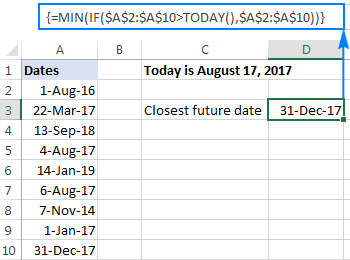#### Get any date closest to today

To get the nearest date in a mixed list of past and future dates, use the classic INDEX MATCH formula with a couple of modifications:

INDEX(range, MATCH(MIN(ABS(range - TODAY())), ABS(range - TODAY()), 0))

Here's how this generic formula works:

• MIN(ABS(range -TODAY())) part. First, you subtract today's date from each date in the range of dates. Then, the ABS function returns the differences as absolute values without regard to their sign. The MIN finds the minimal value, which goes to INDEX MATCH as the lookup value.
• ABS(range -TODAY()) part. You subtract today's date from the range of dates and return an array of absolute values. This array is where INDEX MATCH searches for the lookup value.

In this example, the formula takes the following shape:

`=INDEX(\$A\$2:\$A\$10, MATCH(MIN(ABS(\$A\$2:\$A\$10 - TODAY())), ABS(\$A\$2:\$A\$10 - TODAY()), 0))`

The screenshot below shows the result: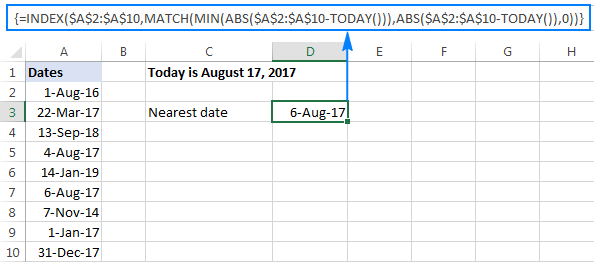Note. All three formulas to get the nearest date are array formulas, so they should be completed by pressing Ctrl + Shift + Enter.

### How to highlight today's date in Excel

When working with a long list of dates or designing your own calendar in Excel, you may want to have the current date highlighted. To have it done, create a conditional formatting rule with this formula:

`=B2=TODAY()`

Where B2 is the left-top-most cell of the range to which the rule applies.

The result may look something similar to this: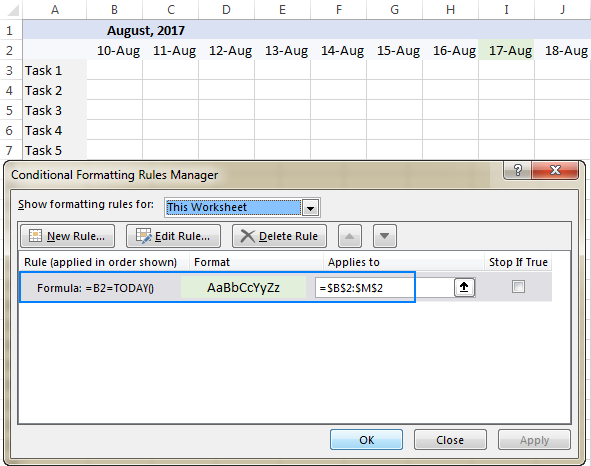The detailed steps to set up a conditional formatting rule can be found here: How to create a conditional formatting rule based on formula.

To have a closer look at the formulas discussed in this tutorial, you are welcome to download our sample workbook below. Thank you for reading!

Excel TODAY formula - examples (.xlsx file)

### More examples of TODAY formula in Excel

For more examples of using the TODAY function in Excel, please check out the following tutorials:

1. Hi there, and thank you very much for all the hints above. Although I have a question:
how could I simply insert this result:
Tue. 22 Feb 2021
this should be semi-automatic. i.e. it should be easy to make a table of such string.

• Hello!
You can apply custom date format:

dddd"." dd mmm yyyy

2. Hello, I have a spreadsheet where there are contract end dates entered in column F. I would like to set up a rule, so that any contract end date that is earlier than TODAY's date is highlighted. It sounds like a simple thing to do but I've looked at different sources and tried setting up some conditional formatting but still haven't managed to make it work for me. Thank you.

3. I know how to use the today function and have been using it successfully for years. Why would one of my workbooks suddenly refuse to calculate any formula tied to the today function? Before you give the obvious answer, I've checked to make sure calculations are set to automatic and have saved/reopened the workbook. Also, all my other formulas are working and, up until a couple of days ago, the today formulas were working as well.

I hope someone has the answer as I use this workbook daily. Thanks.

• Hello!
Unfortunately, without seeing your data it is difficult to give you any advice.
Try opening your file on a different computer. Perhaps the problem is with the date and time settings.

4. Good evening,
I have a Cell A1 which is master cell that filled every day with sum data based on multiple selections from the document and based on Today's date which is in Column B1:B100 A1 is pasted in Column C1:C100 next to today's date and so the next day so that in Column C I have a history of data every day. Something like at the end of the dat Column C cell next to it's date is frozen with the value.

I managed to come this far: =if(B1:B100=today(),\$A\$1,....) and this is it, I can't keep the value frozen as the day passes.

Can anyone help?

• Hello!
I’m sorry but your task is not entirely clear to me. For me to be able to help you better, please describe your task in more detail. Please specify what you were trying to find, what formula you used and what problem or error occurred. Give an example of the source data and the expected result.
It’ll help me understand it better and find a solution for you. Thank you.

5. What is today ?

6. Greetings!
I am creating a spreadsheet, I know how to make today's date appear in a cell and update every day that i open the spreadsheet. I would also like the day of the week to appear in the next cell over that corresponds with the date, which would also update every time i open the spreadsheet. How do i do this? Thank you in advance.

• Hello Josie!
Read the section above carefully - How to insert today's date in Excel. How to determine the day of the week, read here.

7. Hi
please tell me how can get data & time only first time like if I use now() function so it will go to change but I don't want change I just want one timing which time I put text in columns

• Hello Gurpreet!
Replace the formulas with values. Just copy the cell(s) with your date (CTRL+C), then use Paste Special (CTRL+ALT+V) to insert values.

8. I'm working with a spreadsheet to track scholarship. Is there a way for me to have column/cell that updates with the static date of when it was awarded or is going to the field and pressing CTRL+; the only option.

• Hello Mel!
There are two ways to write a static value to a cell: 1. You do it yourself manually. 2. This is done by the program using VBA

9. Hello

I'm using the following formula =(VLOOKUP(MAXIFS(Form1!\$B:\$B,Form1!\$I:\$I,\$B20),Form1!\$B:\$BJ,15,0))
to return a value based on the most recent date (in column b). I now need to return a value from the same column, but for the next most recent date. Basically the values which are being returned are meter readings and I need to be able to return the most recent and the one prior to that so that I can subtract one from the other and calculate a volume.
Would be most grateful for any assistance.

• Hello Stephen!
You use the formula

MAXIFS(Form1!\$B:\$B,Form1!\$I:\$I,\$B20)

to search for the most recent date. Replace it with the formula

LARGE(IF(Form1!\$I:\$I=\$B20,Form1!\$B:\$B,0),1)

To search for the previous date, replace 1 with 2. That is

LARGE(IF(Form1!\$I:\$I=\$B20,Form1!\$B:\$B,0),2)

I hope this will help, otherwise please do not hesitate to contact me anytime.

10. Hi can someone please help. I want a cell to have today's date in, TODAY(), only if another cell is completed. So we only need today's date if a start date has been entered already. Can someone help me with the formular. Many thanks

• Hello Donna!
If I understand your task correctly, the following formula should work for you:

=IF(A1 <> "",TODAY(),"")

I hope this will help

11. Hi,
I need a formula for today date in Excel and if I open the same sheet then Date should remain same as yesterday..

12. Excellent information expressed in the simplest of manners. Thanks.
I am trying to keep track of my savings. I have a current balance, a standard monthly addition and a balance-to-date column. I want the balance-to-date to add the standard monthly addition on the 27th of every month for different savings. Can you assist?

• Hello Jason,

Thank you for your feedback! If you want to increase the value in let's say A1 to a particular amount (1000, for example), you may do it with the help of the following formula:

=IF(TODAY() = DATE(YEAR(TODAY()), MONTH(TODAY()),27), A1+1000,A1)

Or just replace 1000 with the necessary value or cell reference and hit Enter. It'll do the trick.

• Worked like a charm. Thank you very much Alexander! Keep safe.

13. What formula do I use to where it automatically sets the date for that week using a week to week time sheet

• Hello Angel!
For me to be able to help you better, please describe your task in more detail. It’ll help me understand it better and find a solution for you. Thank you.

14. Hi I am looking for a formula for:

If I have a dates in column A. Column B, sometimes it is either left blank or has a date.
I want to populate a formula that shows how long from today's date OR if there's a date in Column B, therefore it should choose that date in Column B, but not today's date, so if Column B had no date, it will choose today's date.

Is that possible?

• Hello Pham!
=IFERROR(IF(B1>A1, B1-A1, TODAY()-A1), TODAY()-A1)

15. When i insert =today() formula date come yesterday.excel change date in day time 12pm.i check my region in cell and formate of time date all are correct.but in Excel or google sheet have problem.have any solution?i am thankful to you for answering me.

16. I want a cell to default to the current date that row was completed. If I use TODAY(), it changes every day. Row 1 would be completed on 1 day, row 2 completed on another day. The only way the date would ever change is if that row was modified. Thanks in advance

17. =Today() default format(mm/dd/yyy) can be changed to dd/mm/yyyy?

18. How do I do an age analysis on Excel?
Created Date Time Age Analyse (TODAY)
21/06/2019 12:19

19. hello experts,
is there any formula to get the monthly date from cell A1 to A30/31?
for example:
A1= 1/10/2019
A2=2/10/2019
.
A31 =31/10/2019

• Hi Chanu,

It sounds like Excel's AutoFill feature is exactly what you are looking for. For more information, please see How to auto fill dates in Excel.

20. Hi, how to make a formula that counts the items that have yesterday and today's date?

21. I am attempting to use the formula as indicated above, however the result is displayed as #VALUE!
I am using the following string:
=MIN(IF(\$E\$5:\$E\$9>TODAY(),\$E\$5:\$E\$9))
Any idea where I am going wrong?

22. I'd like excel to calulate the time between two dates to show how long someone was on a waiting list but when the second date is blank I'd like excel to calculate using today's date. I can do those two functions separately but not sure how to do them both together?

23. Hello, can anyone please help. I have a list of renewal dates and I’m looking for an excel formula which will highlight in yellow, the dates which are less than 1 month from today. Thank you

24. Hi,
I'm always looking at prior day and getting it =TODAY()-1. How can I get the Fridays date on Monday by using TODAY() formula?

25. Hi,
I need like this formula,
D36 is 15-Aug-2019
=IF(AND(D36>TODAY(), AND((=TODAY()-D36)>=0, (=TODAY()-D36)<=10))),"Inside","Outside")
When i use this one getting error, so please explain where i did mistake?
thank you

26. I WOULD TO HAVE ACCESS TO POWER PIVOT,POWER QUERRY COURSE,POWER B TUTORIAL

27. how to freeze =now() or =today()? It must not change when we entered anywhere in cells
=if(A2="",today(),"1")

28. Hi I am using the TODAY formula in a roster to highlight the current date on a roster however it is highlighting the incorrect date, the date highlighted is two days ahead of the correct date.

29. Hello- can really use help with this.....I have a list of assets in one column with just a number representing the value of how old each asset is.....I need a formula for another column- YEAR BUILT- so I need a YEAR formula that I can combine with TODAY()......I just can't figure out how to do it....I need to use TODAY(), because this would be an ongoing list

30. Hi

If I input on a cell number '1' then the current date will show, if '0' the cell leave blank.

Thanks for help

31. If have =+TODAY()+1 in cell that will give me tomorrow date, I want it will stop automatically when month will be over on 30th or 31st

32. Hopefully someone will be able to tell me what i am doing wrong here. I have created a seperate worksheet that shows data for each day of the year as either "a" or "u". I'm wanting to create a formula that will count the number of occurences of "u" in the next 7 days and the next 30 days. Each formula I attempt throws an error as i can't figure a way to create a date range using todays date as the start point.
I started with the below for counting out to 7 days
=COUNTIFS('Calendar'!\$2:\$2,TODAY()+7,'Response'!3:3,"U") or =COUNTIFS('Calendar'!\$2:\$2,TODAY():TODAY()+7,'Response'!3:3,"U")

Hopefully someone can help me. Thanks!!

33. Hello, thanks for the post! It is very informative.
I am working on a budget spreadsheet and I am trying to find the correct syntax for an IF ELSE formula for Excel. Here is my formula:
IF(TODAY()>06/01/2019,G10-G11,"Not EOM")
The goal is to have the program give the difference between income (G10) and expenses (G11) AFTER the end of the month. It doesn't seem to be recognizing the ">" sign. For it gives G10-G11. Can you help? Thanks!

• Not sure if your question was ever answered, but you could consider using the number format of the date, in your case 43471. This is how excel uses dates in calculations, and so may aid you.
i.e.
IF(TODAY()>43471,G10-G11,"Not EOM")

Cheers,
D

34. Cell A2 =TODAY(), the data in cells B2:G2 fail to move down 1 row to A3 when A2's date is updated. I would also like the formatting from below to automatically be applied to B2:G2 as the new row is created. Thank you.

35. Hello,
if i enter in cell name of the task, the other cell need to show the date.

36. Can somebody help me how to get the date of loyalty of employee in 10 years, example supposing he/she was hired March 03, 2008 then he got received loyalty award at March 03, 2018. This date will show in cell. Then if he/she 15 years of received he/she will received again loyalty award of 5 years. The date will also show when he/she will received that award. Thanks in advance. This is a great help for me! God bLess :)

37. Hi Svetlana,
Was wondering if there is a way to have excel change the year of a date entered. For example I enter 5/29 (and I have formatted cells to the 03/14/01 format), so excel returns 05/29/19. But I want a formula so that it returns 05/29/18. So same date just for last year?
Thank you very much for your help. Love your name by the way.

38. I have a list of events in column A with completion dates in column B. I use this formula to find out how many events have not been completed in one (1) year:
=COUNTIF(B2:B202,"<2/4/2018")
I manually put in the date as today's month and day and last year. Is there a way I can have Excel do that so it is always up to date without me having to manually entering the date? I tried this but it does not work: =COUNTIF(C2:C202,"<TODAY()-365")

Thank you,
Bob

39. @ Svetlana Cheusheva: Thanks for saving a lots of time.

40. I have the TODAY function in cell A1. I want cell G9 to update to the previous month/year. Ex. Today's date is 1/11/2019 in cell A1 so I want G9 to show December 2018. And then when A1 shows 2/1/19, I want G9 to show January 2019. Does a function and/or condition exist for this?

41. Guys,
I have all the dates for the year in column A starting in cell A2 from 01-Jan-19 through to 31-Dec-19. I used your guidance to create a rule to highlight the current day (thanks). I would like to create a rule so that all of the days that are now past would be in a gray text colour, whereas the current date and future dates remain in a black font colour. Any advice much appreciated.

• =C1:C99<TODAY()-30

this has highlighted for me the dates that are 30 days past from today. I use this in my outreach sheet to keep relationships current

42. How do I auto fill just the current Month?Like December, January or February in a cell every time I open the document? I am making forms that I only want the month not today's date

• Hello, Jim:
To enter the current month in a cell enter this formula in the cell:
=TEXT(TODAY(),"mmmm")

43. Hi,

How do you add the time to the Excel formula for today's date? Using the formula,

=CONCATENATE("Today is: ",TEXT(TODAY(), "dd mmmm yyyy hh:mm:ss"))

I get "Today is: 25 October 2018 00:00:00" -- and not the current time, no matter what time it is. Even after reading many, many, websites on this topic, I am still confused (or rather, more confused), and I'm not really sure how the time stamp can still be added.

Thanks and much appreciated,
R

• Use NOW(), not TODAY(). TODAY() is only the date, NOW() includes the time.

44. Dear Everyone

May anyone can help me please
i would like to have a function that when we key in today it will be show date today this date will be not change since we already move to next day..
or When we key in on one cell date today will show automatically.

Thank you so much

45. I have a column of dates with some empty cells - how can i fill in all the empty cells with today's DATE?

• Mandi:
TODAY() will give you today's date. If you enter this function in a cell, the cell will always display the current date.

46. HI Steve
can you suggest me a formula, where i need current date in date coloum if i make any change in that particular Row.

6-May-18 . Linson N/A N/A 20-Jun-17 20-Jun-17

so if make any change in any details by previous date (6-May-18) should become current date.

• Linson:
I believe if you want a cell to update based on change to another cell it requires some code. In this case I think you could use some VBA, but VBA is not in this blog's frame. If you wanted to tackle this you could google "excel update cell if another cell changes".

47. Hello Svetlana, where you're from, Ukraine or Russia? :)
Nice job!
Thanks you dear...

• Thank you, Louis. I'm from Belarus :)

• Thanks its help me a lot

48. THANK YOU. A million times, THANK YOU!!!

49. Steve:
Enter this into cell B2 adjacent to cell A2
=IF(ISNUMBER(A2),(TODAY()+21),"")
then copy the formula down the B column.

50. Can you help please? I have a spreadsheet on which I want to do the following: if I enter a number in a cell in Col A I want the adjacent cell in Col B to return a date which is today plus 21 days. If no number is entered in a cell in Col A I want the adjacent cell in Col B to remain blank. So:
Col A Col B
2 today's date + 21 days is returned

3 today's date + 21 days is returned
1 today's date + 21 days is returned

• Hi Steve,

Supposing your data begins in row 2, you enter the following formula in B2, and then copy it down the column:
=IF(ISNUMBER(A2), TODAY()+21, "")

• Thanks you much , its more useful.

• Can we get a trend of values based on today fuction.I am using the todays value to divide expenses to get the average expenses daily